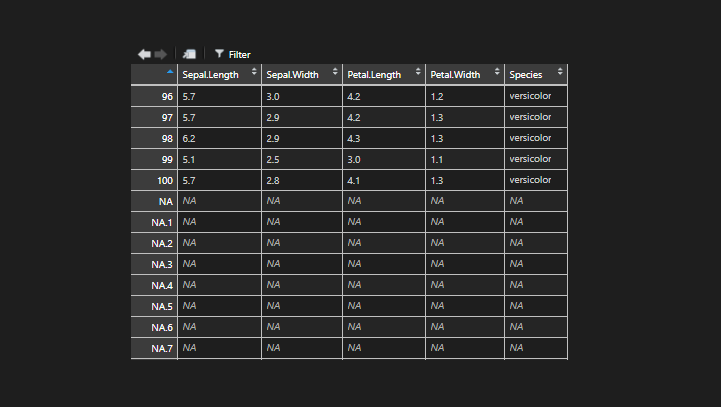# NA row names in R data frameHere is one of the ways how you can run into this problem and get NA row names in the R data frame.

If you’re doing some data wrangling and create new versions of the same data frame, it is easy to get confused and use a strange or accidental combination in the same function. That can lead to normal data frame row numbering in combination with NA.

Here is an example of the iris dataset.

First, there is a dataset as a copy of iris. Then new data frame with subset. The problem appears in the next subset, where criteria from the first data frame are used, which is larger and with different row content.

```df <- iris

new_df <- subset(iris, iris\$Species == 'versicolor')

# mistakenly used df column Sepal.Length
new_df <- subset(new_df, df\$Sepal.Length < 6)```

R allows you to do that, and there is no problem in that if it is what you wanted.

In case your problem is solved, and you want to know some useful RStudio tips, I recommend this post: My favorite RStudio tips and tricks.